# (AAAI2020 Yao) Graph Few-shot Learning via knowledge transfer

22-5-13 seminar上和大家分享了这篇文章，重新整理一下在博客里备个份
Graph few-shot learning via knowledge transfer

## 前置知识

### 小样本学习简介

#### 小样本学习的训练流程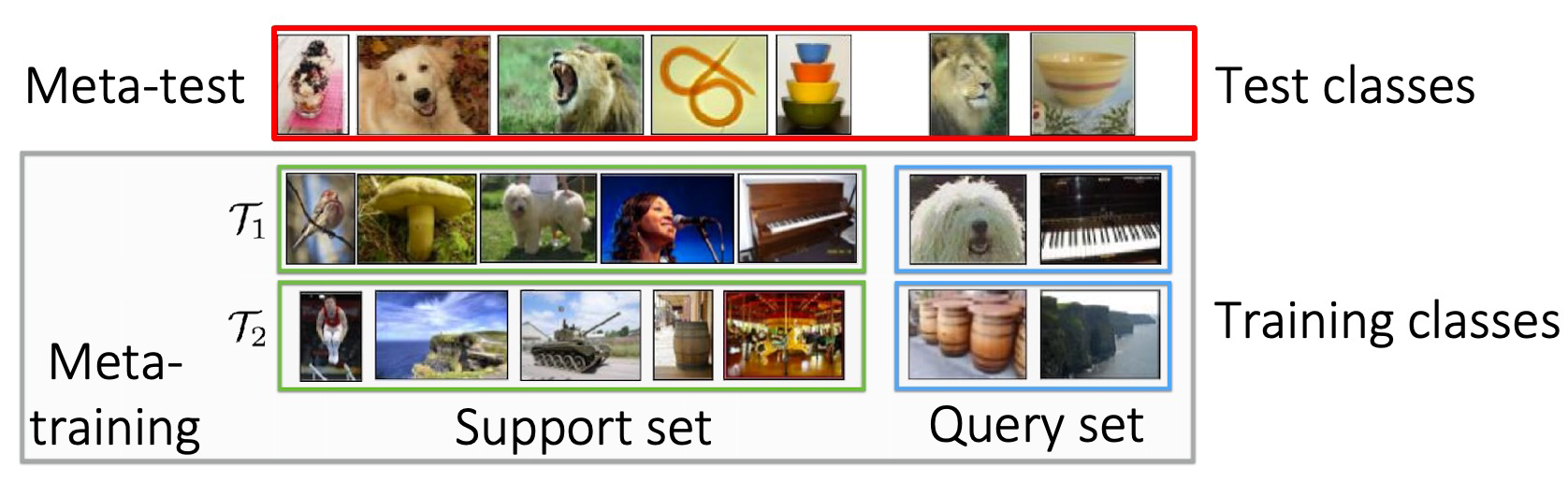way，指的是数据集中类别的数量，图中为5个类别。shot，指的是数据集中每个类别图片的数量，图中每个类别只有一张图片。

#### 基于度量的方法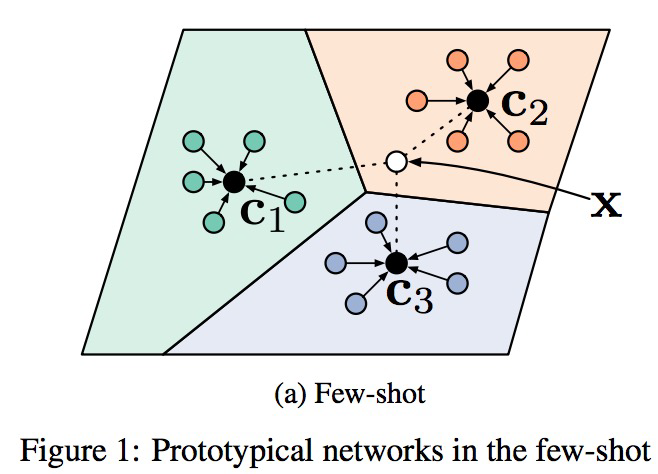#### 基于梯度/优化的方法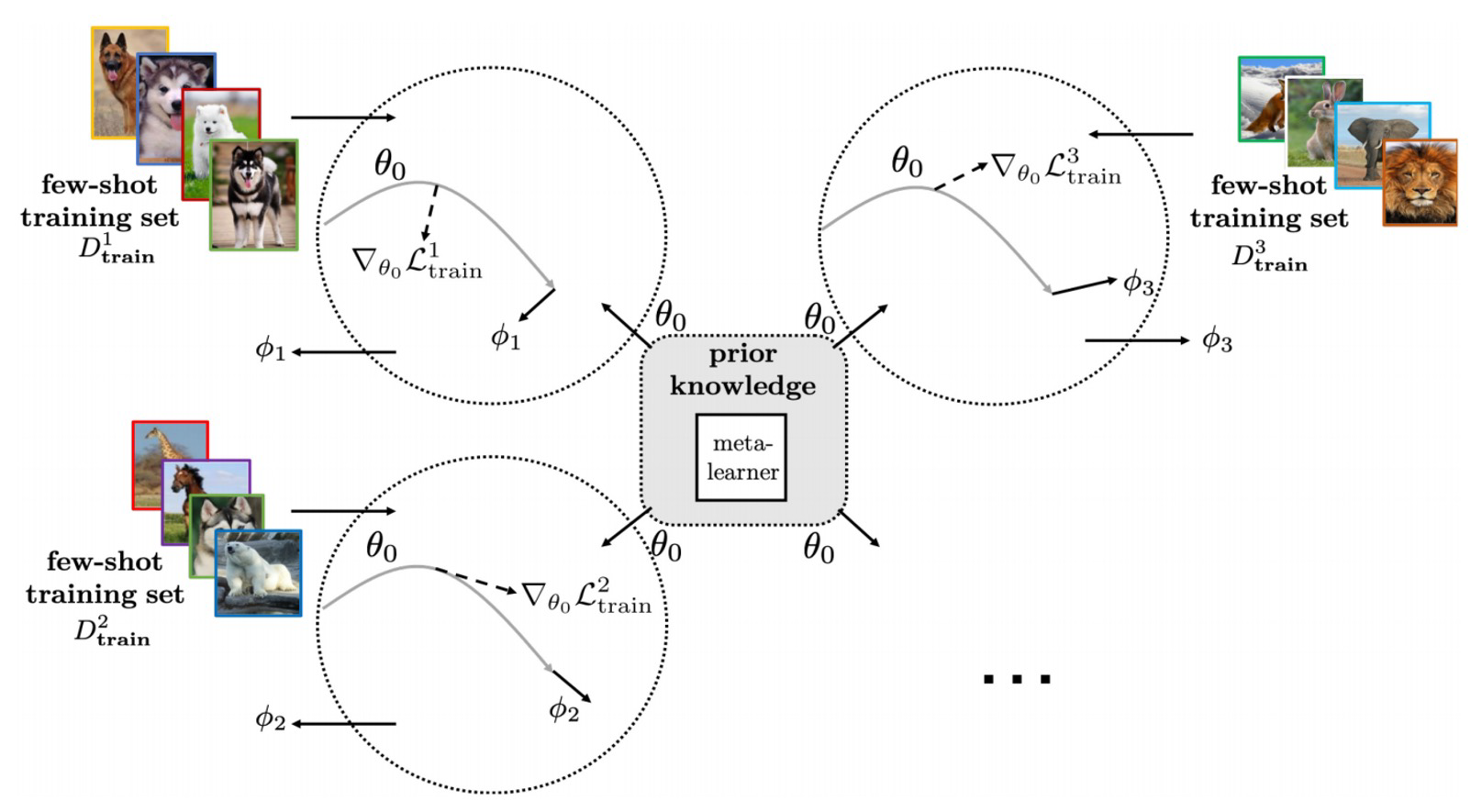## 本文内容

### Method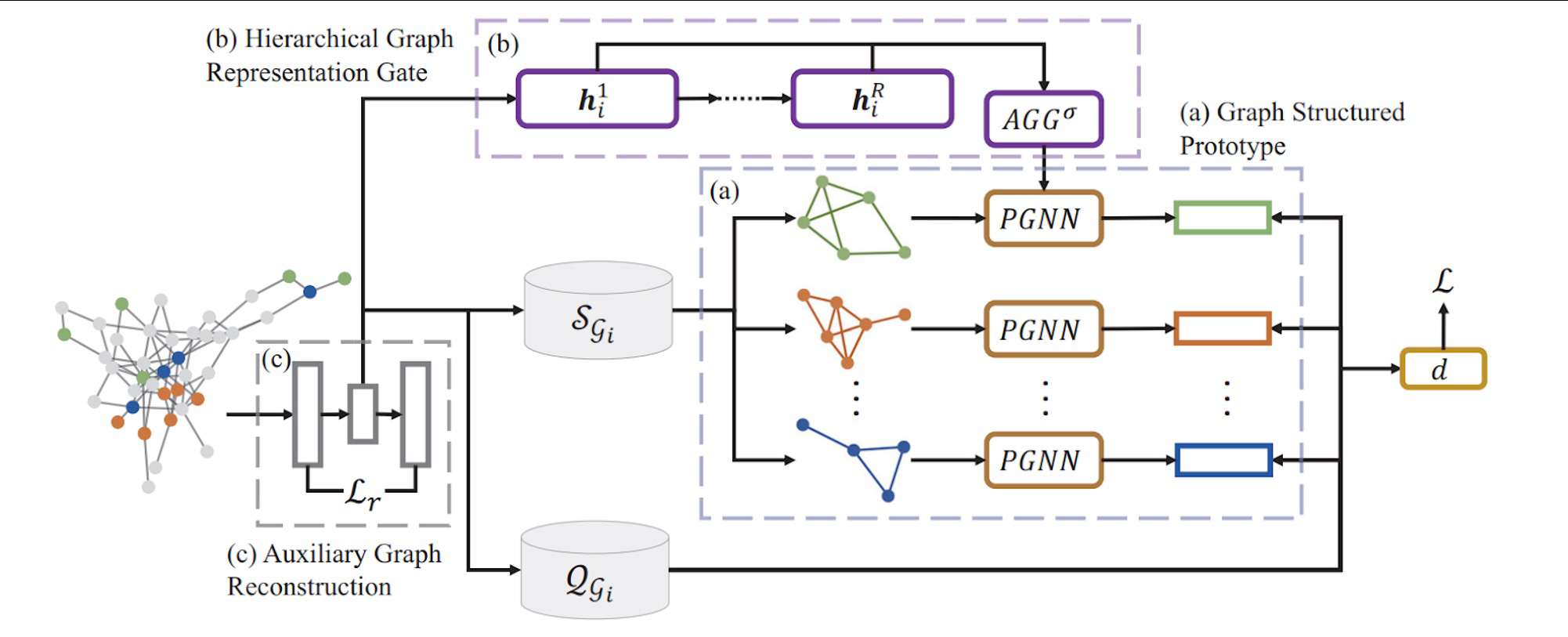### step a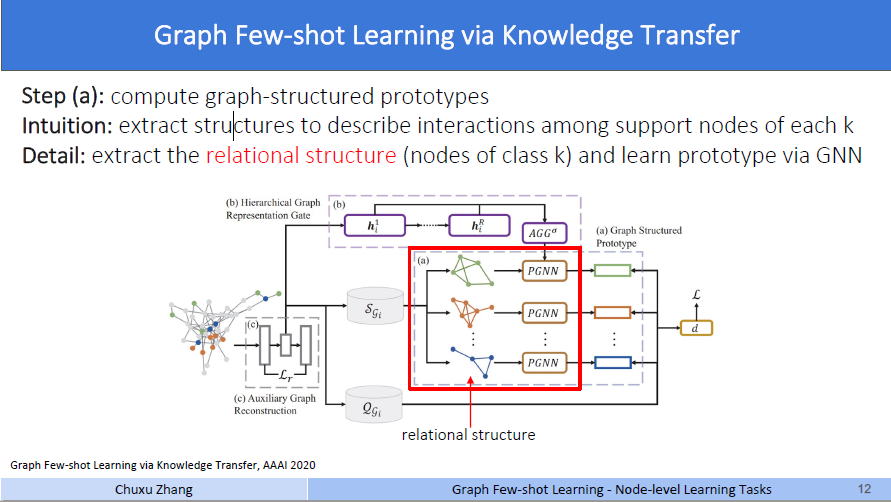$\mathbf{c}_{i}^{k}=\operatorname{Pool}_{j=1}^{n_{i}^{k}}\left(\operatorname{PGNN}_{\phi}\left(\mathcal{R}_{i}^{k}, f_{\theta}\left(\mathcal{S}_{i}^{k}\right)\right)[j]\right)$

### step b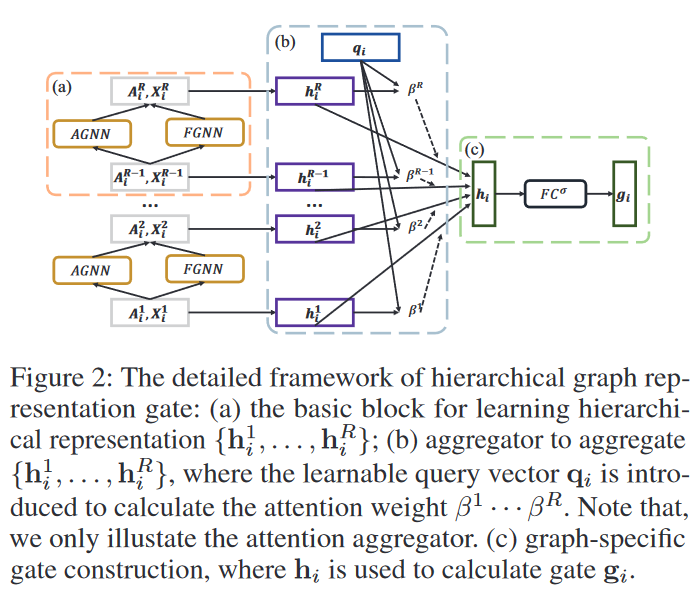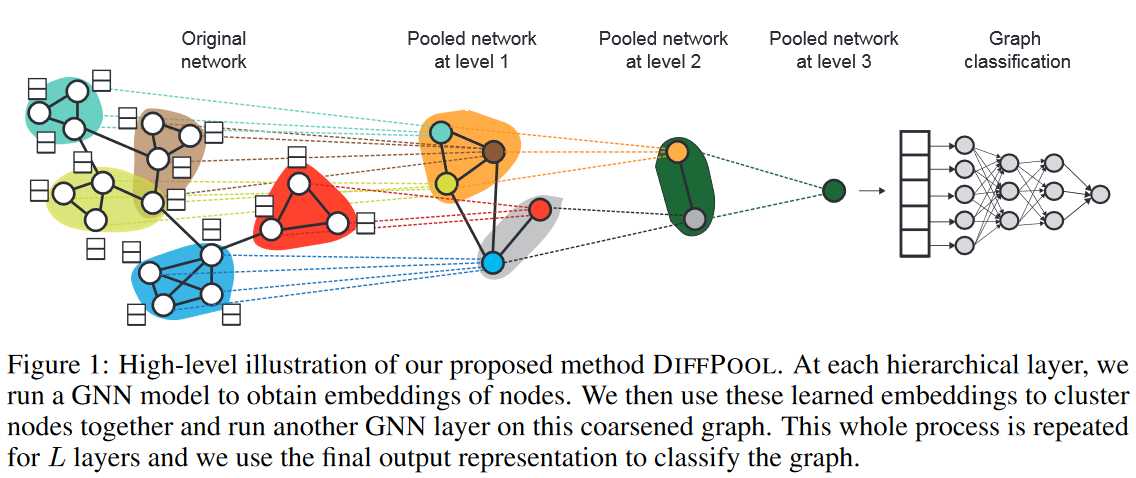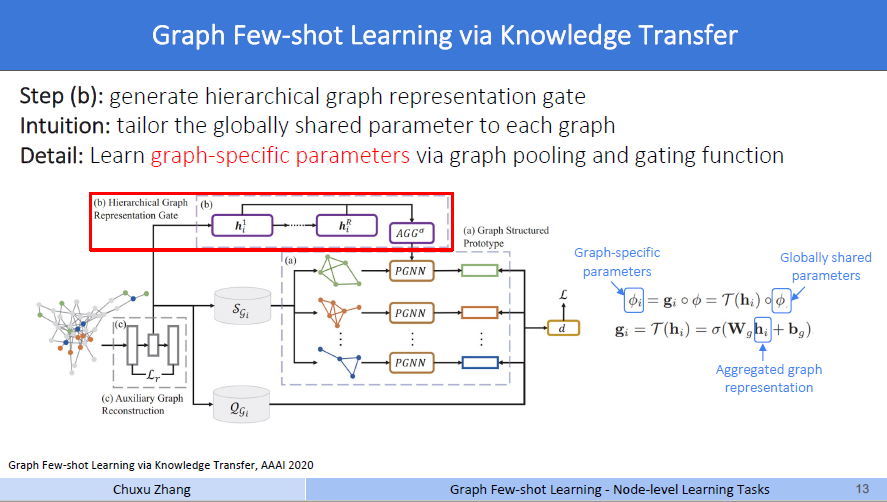### step c

$\mathcal{L}_{i}^{k}=-\sum_{\left(\mathbf{x}_{i, j}^{q_{i}}, y_{i, j}^{q_{i}}\right) \in \mathcal{Q}_{i}^{k}} \log \frac{\exp \left(-d\left(f_{\theta}\left(\mathbf{A}, \mathbf{x}_{i, j}^{q_{i}}\right), \mathbf{c}_{i}^{k}\right)\right)}{\sum_{k^{\prime}} \exp \left(-d\left(f_{\theta}\left(\mathbf{A}, \mathbf{x}_{i, j}^{q_{i}}\right), \mathbf{c}_{i}^{k^{\prime}}\right)\right)},$

$\mathcal{L}_{r}\left(\mathbf{A}_{i}, \mathbf{X}_{i}\right)=\left\|\mathbf{A}_{i}-\mathrm{GNN}_{d e c}\left(\mathbf{Z}_{i}\right) \mathrm{GNN}_{d e c}^{T}\left(\mathbf{Z}_{i}\right)\right\|_{F}^{2},$

Zi就是一个编码器，也就是一个GNN，生成图中每个节点的表示，然后通过一个解码器，在乘以其转置，得到的这个结果用于描述图的结构，和邻接矩阵相减做F范数。$\min _{\Theta} \sum_{i=1}^{N_{t}} \mathcal{L}_{i}+\gamma \mathcal{L}_{r}\left(\mathbf{A}_{i}, \mathbf{X}_{i}\right)$

### Algorithm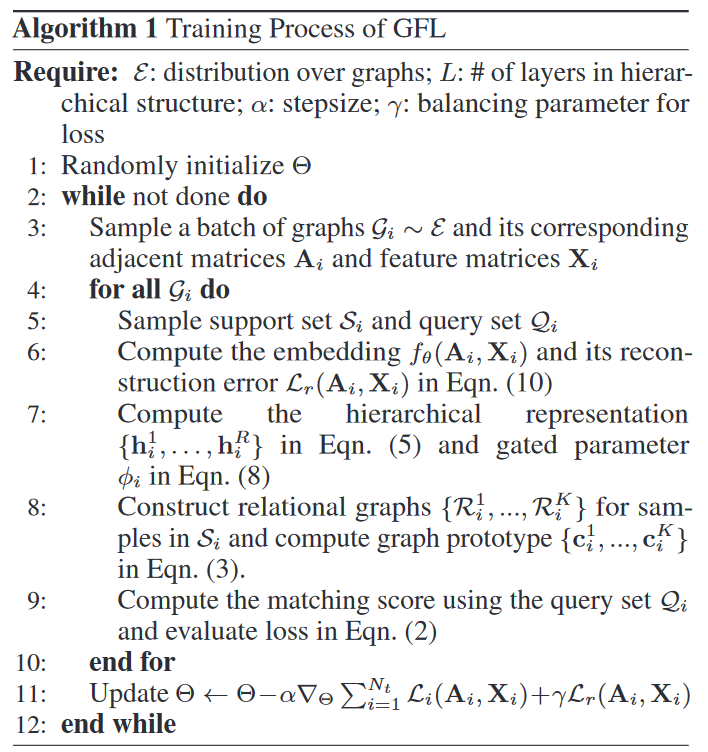1. 初始化参数Θ，
2. 采样一些图，得到领接矩阵和特征矩阵
3. 对于每一个图，采样出支持集和查询集
4. 计算embedding和重构损失Lr stepc中的内容
5. 计算这个图的图表示，和参数φ做门控 stepb中的内容
6. 构建关系图并计算图原型
7. 计算图原型和查询集中embedding的距离，并构成损失函数Li
8. 反向传播更新参数

## experiment

### setting### result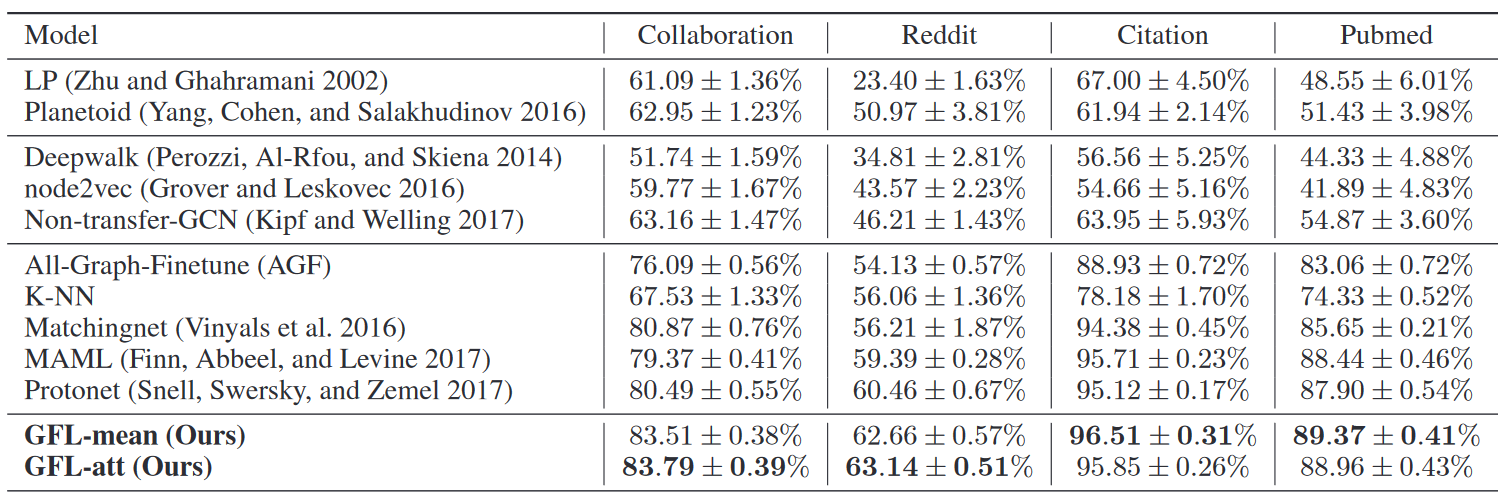KNN往上都传统的图神经网络方法，可以看到效果都不咋样。在Meta-train阶段只有100多个甚至几十个节点训练出来的模型，在剩下的查询集上表现糟糕是理所应当的。

## 对我的启发

 Snell J, Swersky K, Zemel R. Prototypical networks for few-shot learning[J]. Advances in neural information processing systems, 2017, 30.
 Vinyals O, Blundell C, Lillicrap T, et al. Matching networks for one shot learning[J]. Advances in neural information processing systems, 2016, 29.
 Finn C, Abbeel P, Levine S. Model-agnostic meta-learning for fast adaptation of deep networks[C]//International conference on machine learning. PMLR, 2017: 1126-1135.
 Ying Z, You J, Morris C, et al. Hierarchical graph representation learning with differentiable pooling[J]. Advances in neural information processing systems, 2018, 31.

posted @ 2022-05-14 22:28  lynk  阅读(31)  评论(0编辑  收藏  举报